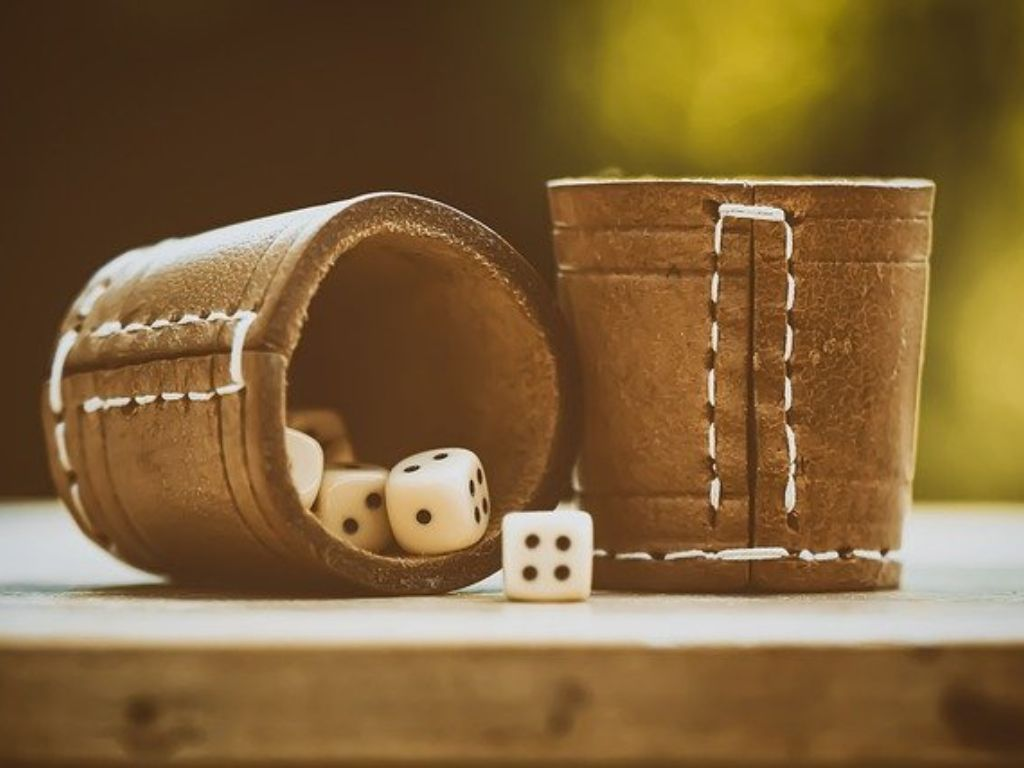## Probability Class 10th

Introduction In mathematics, there are many branches and among all, probability is a very interesting branch. In class 9th, we studied probability based on real-life experiments, which is known as experimental probability. We noticed that real-life experiments are helpful to get the theoretical probability. In class 10th, we shall study theoretical probability which is very …